Examples

Chapter 4 Class 12 Determinants
Serial order wise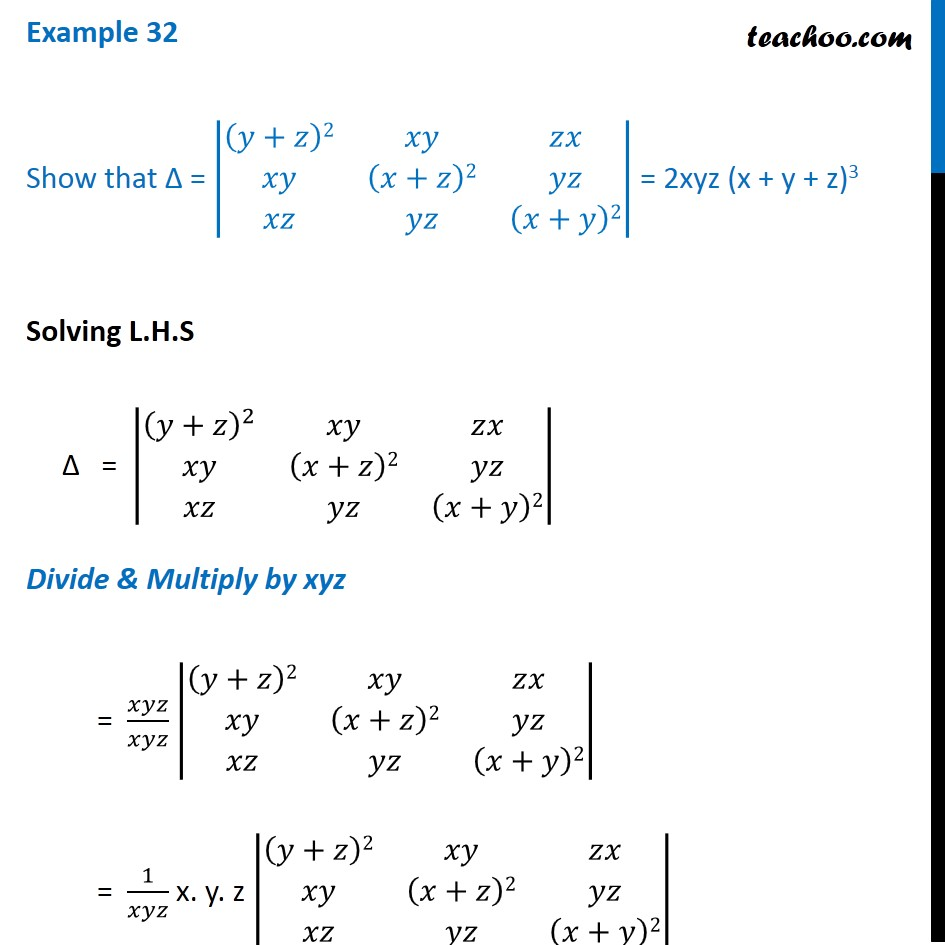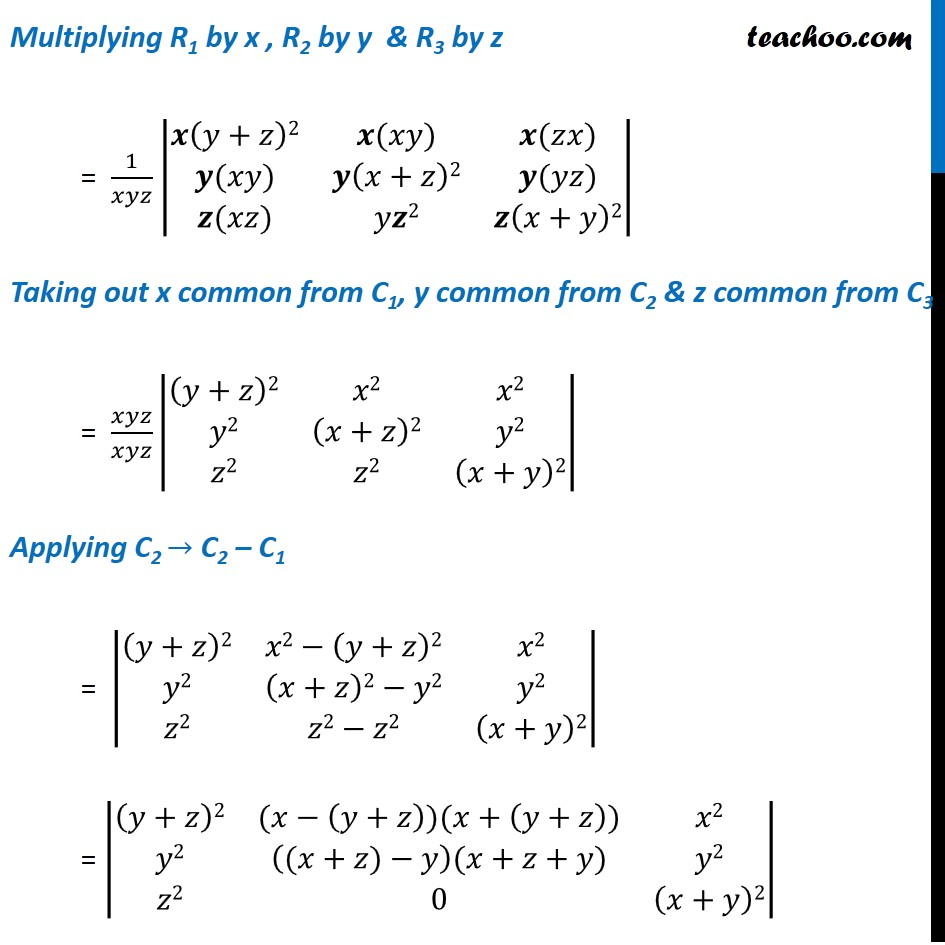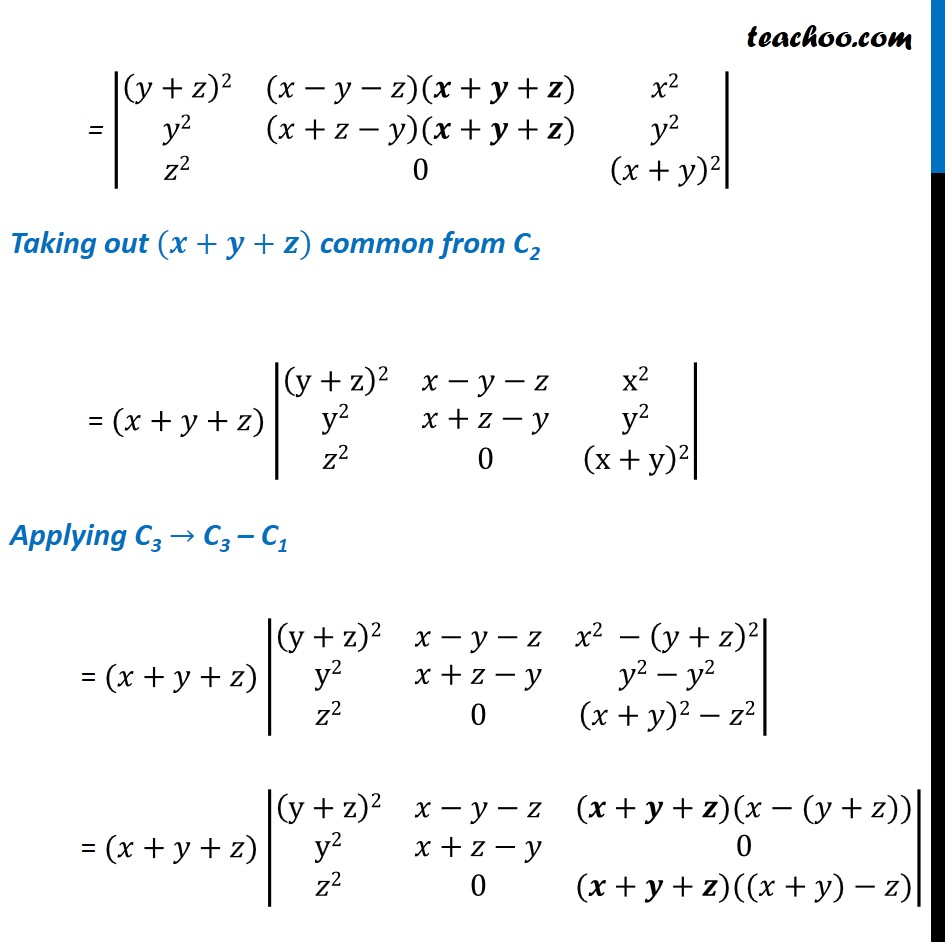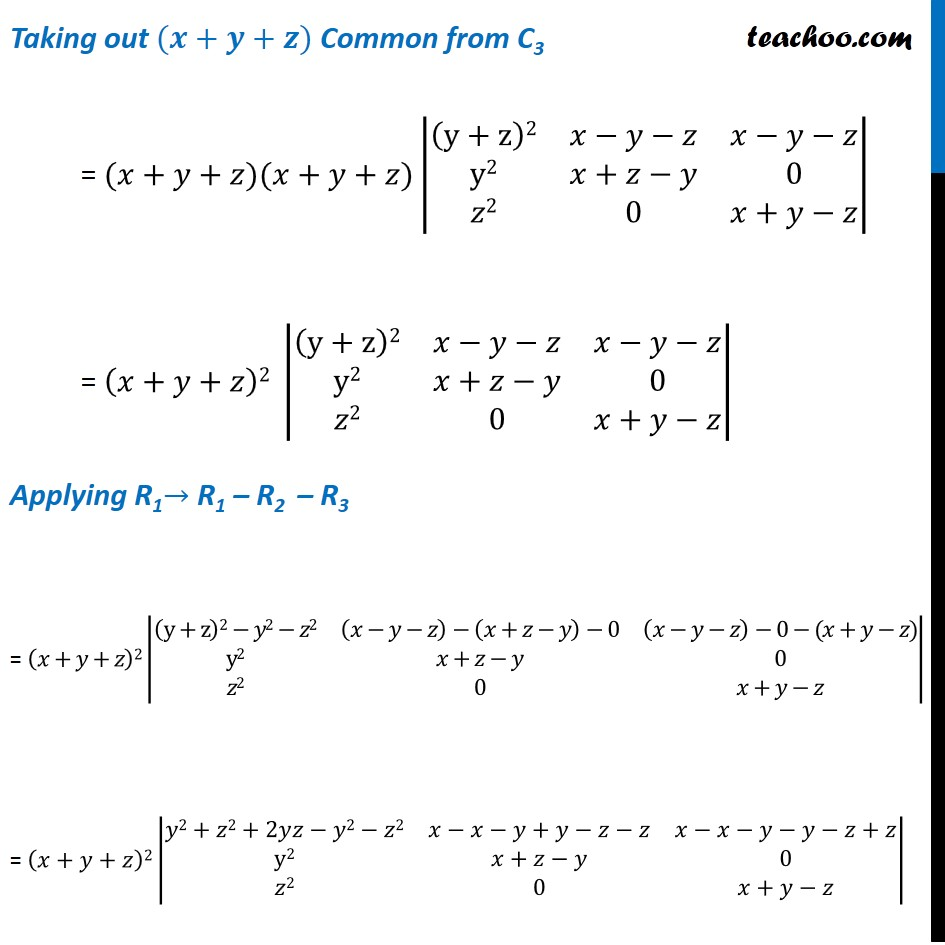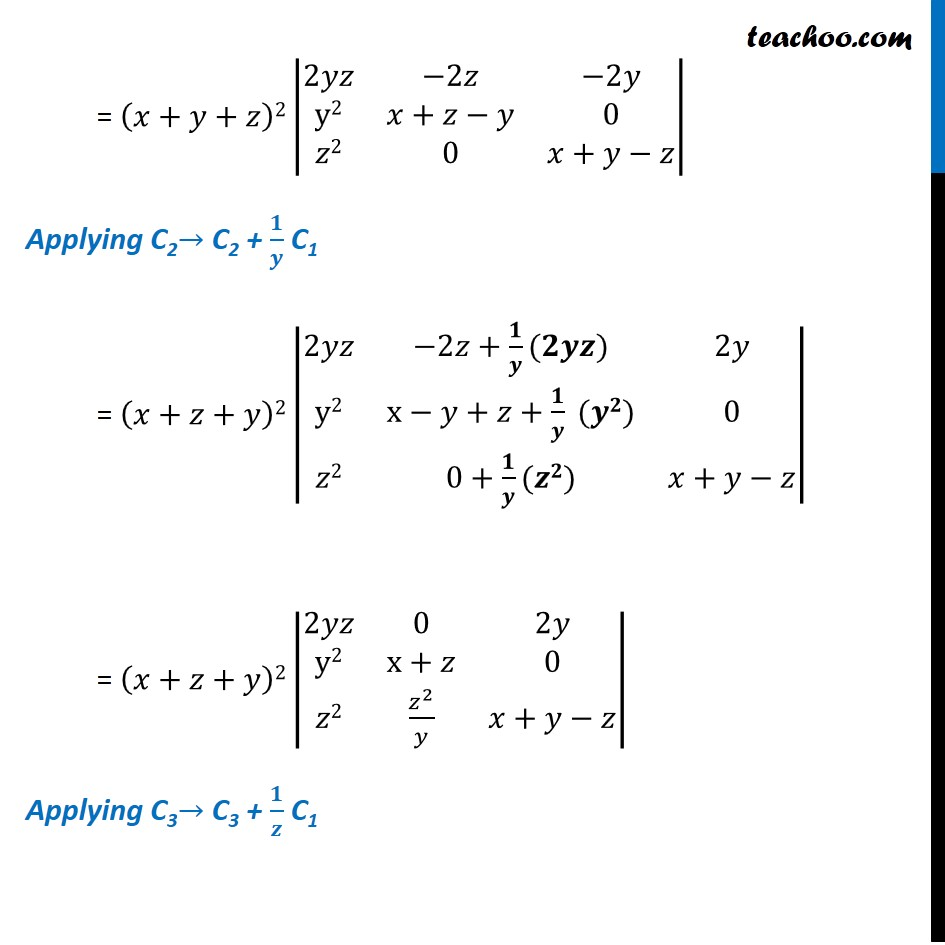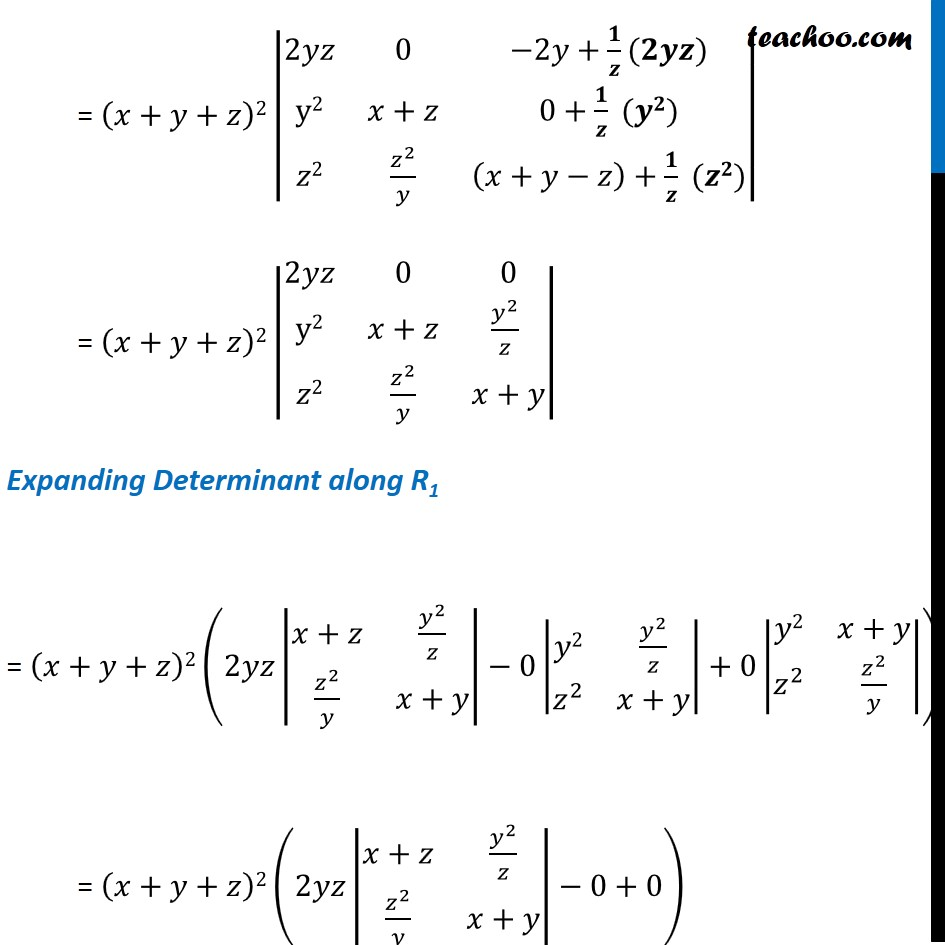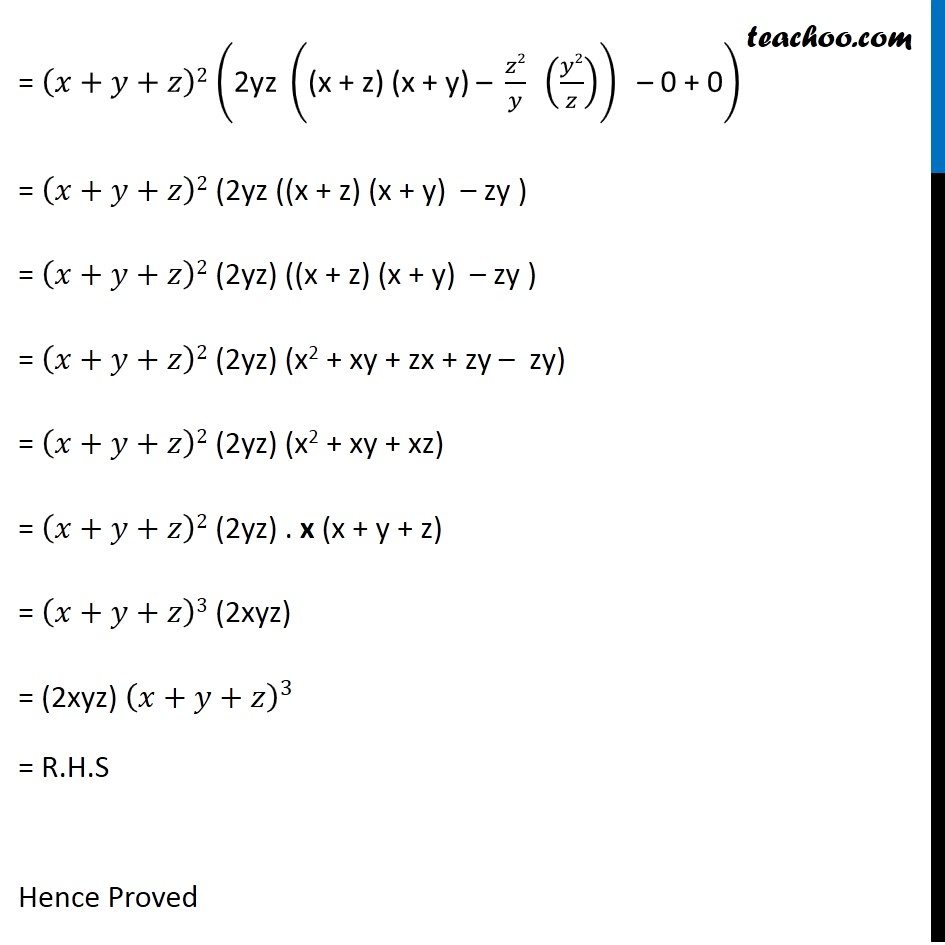Learn in your speed, with individual attention - Teachoo Maths 1-on-1 Class

### Transcript

Question 14 Show that Δ = |■8((𝑦+𝑧)2&𝑥𝑦&𝑧𝑥@𝑥𝑦&(𝑥+𝑧)2&𝑦𝑧@𝑥𝑧&𝑦𝑧&(𝑥+𝑦)2)| = 2xyz (x + y + z)3 Solving L.H.S Δ = |■8((𝑦+𝑧)^2&𝑥𝑦&𝑧𝑥@𝑥𝑦&(𝑥+𝑧)2&𝑦𝑧@𝑥𝑧&𝑦𝑧&(𝑥+𝑦)2)| Divide & Multiply by xyz = 𝑥𝑦𝑧/𝑥𝑦𝑧 |■8((𝑦+𝑧)2&𝑥𝑦&𝑧𝑥@𝑥𝑦&(𝑥+𝑧)2&𝑦𝑧@𝑥𝑧&𝑦𝑧&(𝑥+𝑦)2)| = 1/𝑥𝑦𝑧 x. y. z |■8((𝑦+𝑧)2&𝑥𝑦&𝑧𝑥@𝑥𝑦&(𝑥+𝑧)2&𝑦𝑧@𝑥𝑧&𝑦𝑧&(𝑥+𝑦)2)| Multiplying R1 by x , R2 by y & R3 by z = 1/𝑥𝑦𝑧 |■8(𝒙(𝑦+𝑧)2&𝒙(𝑥𝑦)&𝒙(𝑧𝑥)@𝒚(𝑥𝑦)&𝒚(𝑥+𝑧)2&𝒚(𝑦𝑧)@𝒛(𝑥𝑧)&𝑦𝒛2&𝒛(𝑥+𝑦)2)| Taking out x common from C1, y common from C2 & z common from C3 = 𝑥𝑦𝑧/𝑥𝑦𝑧 |■8((𝑦+𝑧)2&𝑥2&𝑥2@𝑦2&(𝑥+𝑧)2&𝑦2@𝑧2&𝑧2&(𝑥+𝑦)2)| Applying C2 → C2 – C1 = |■8((𝑦+𝑧)2&𝑥2−(𝑦+𝑧)2&𝑥2@𝑦2&(𝑥+𝑧)2−𝑦2&𝑦2@𝑧2&𝑧2−𝑧2&(𝑥+𝑦)2)| = |■8((𝑦+𝑧)2&(𝑥−(𝑦+𝑧))(𝑥+(𝑦+𝑧))&𝑥2@𝑦2&((𝑥+𝑧)−𝑦)(𝑥+𝑧+𝑦)&𝑦2@𝑧2&0&(𝑥+𝑦)2)| = |■8((𝑦+𝑧)2&(𝑥−𝑦−𝑧)(𝒙+𝒚+𝒛)&𝑥2@𝑦2&(𝑥+𝑧−𝑦)(𝒙+𝒚+𝒛)&𝑦2@𝑧2&0&(𝑥+𝑦)2)| Taking out (𝒙+𝒚+𝒛) common from C2 = (𝑥+𝑦+𝑧)|■8((y+z)2&𝑥−𝑦−𝑧&x2@y2&𝑥+𝑧−𝑦&y2@𝑧2&0&(x+y)2)| Applying C3 → C3 – C1 = (𝑥+𝑦+𝑧)|■8((y+z)2&𝑥−𝑦−𝑧&𝑥2 −(𝑦+𝑧)2@y2&𝑥+𝑧−𝑦&𝑦2−𝑦2@𝑧2&0&(𝑥+𝑦)2−𝑧2)| = (𝑥+𝑦+𝑧)|■8((y+z)2&𝑥−𝑦−𝑧&(𝒙+𝒚+𝒛)(𝑥−(𝑦+𝑧))@y2&𝑥+𝑧−𝑦&0@𝑧2&0&(𝒙+𝒚+𝒛)((𝑥+𝑦)−𝑧))| Taking out (𝒙+𝒚+𝒛) Common from C3 = (𝑥+𝑦+𝑧)(𝑥+𝑦+𝑧)|■8((y+z)2&𝑥−𝑦−𝑧&𝑥−𝑦−𝑧@y2&𝑥+𝑧−𝑦&0@𝑧2&0&𝑥+𝑦−𝑧)| = (𝑥+𝑦+𝑧)2 |■8((y+z)2&𝑥−𝑦−𝑧&𝑥−𝑦−𝑧@y2&𝑥+𝑧−𝑦&0@𝑧2&0&𝑥+𝑦−𝑧)| Applying R1→ R1 – R2 – R3 = (𝑥+𝑦+𝑧)2|■8((y+z)2−𝑦2−𝑧2&(𝑥−𝑦−𝑧)−(𝑥+𝑧−𝑦)−0&(𝑥−𝑦−𝑧)−0−(𝑥+𝑦−𝑧)@y2&𝑥+𝑧−𝑦&0@𝑧2&0&𝑥+𝑦−𝑧)| = (𝑥+𝑦+𝑧)2|■8(𝑦2+𝑧2+2𝑦𝑧−𝑦2−𝑧2&𝑥−𝑥−𝑦+𝑦−𝑧−𝑧&𝑥−𝑥−𝑦−𝑦−𝑧+𝑧@y2&𝑥+𝑧−𝑦&0@𝑧2&0&𝑥+𝑦−𝑧)| = (𝑥+𝑦+𝑧)2|■8(2𝑦𝑧&−2𝑧&−2𝑦@y2&𝑥+𝑧−𝑦&0@𝑧2&0&𝑥+𝑦−𝑧)| Applying C2→ C2 + 𝟏/𝒚 C1 = (𝑥+𝑧+𝑦)2|■8(2𝑦𝑧&−2𝑧+𝟏/𝒚(𝟐𝒚𝒛)&2𝑦@y2&x−𝑦+𝑧+𝟏/𝒚 (𝒚𝟐)&0@𝑧2&0+𝟏/𝒚(𝒛𝟐)&𝑥+𝑦−𝑧)| = (𝑥+𝑧+𝑦)2|■8(2𝑦𝑧&0&2𝑦@y2&x+𝑧&0@𝑧2&𝑧^2/𝑦&𝑥+𝑦−𝑧)| Applying C3→ C3 + 𝟏/𝒛 C1 = (𝑥+𝑦+𝑧)2|■8(2𝑦𝑧&0&−2𝑦+𝟏/𝒛(𝟐𝒚𝒛)@y2&𝑥+𝑧&0+𝟏/𝒛 (𝒚𝟐)@𝑧2&𝑧^2/𝑦&(𝑥+𝑦−𝑧)+𝟏/𝒛 (𝒛𝟐))| = (𝑥+𝑦+𝑧)2|■8(2𝑦𝑧&0&0@y2&𝑥+𝑧&𝑦^2/𝑧 @𝑧2&𝑧^2/𝑦&𝑥+𝑦)| Expanding Determinant along R1 = (𝑥+𝑦+𝑧)2(2𝑦𝑧|■8(𝑥+𝑧&𝑦^2/𝑧@𝑧^2/𝑦&𝑥+𝑦)|−0|■8(𝑦2&𝑦^2/𝑧@𝑧^2&𝑥+𝑦)|+0|■8(𝑦2&𝑥+𝑦@𝑧^2&𝑧^2/𝑦)|) = (𝑥+𝑦+𝑧)2(2𝑦𝑧|■8(𝑥+𝑧&𝑦^2/𝑧@𝑧^2/𝑦&𝑥+𝑦)|−0+0) = (𝑥+𝑦+𝑧)2 ("2yz " ("(x + z) (x + y) – " 𝑧2/𝑦 " " (𝑦2/𝑧))" – 0 + 0" ) = (𝑥+𝑦+𝑧)2 (2yz ((x + z) (x + y) – zy ) = (𝑥+𝑦+𝑧)2 (2yz) ((x + z) (x + y) – zy ) = (𝑥+𝑦+𝑧)2 (2yz) (x2 + xy + zx + zy – zy) = (𝑥+𝑦+𝑧)2 (2yz) (x2 + xy + xz) = (𝑥+𝑦+𝑧)2 (2yz) . x (x + y + z) = (𝑥+𝑦+𝑧)3 (2xyz) = (2xyz) (𝑥+𝑦+𝑧)^3 = R.H.S Hence Proved